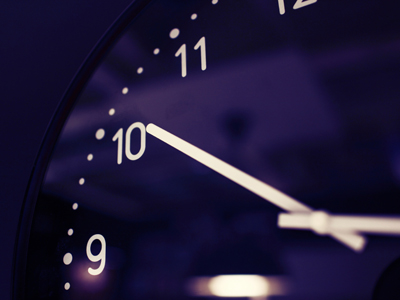What power needs to be added to 10 to give it a value of a million?

# Level 7-8 Algebra - Powers

Remember those pesky little numbers called powers that turned out to be powerful little numbers? I'm sure you do - they play a key role in KS3 Maths, especially when working on algebra.

Powers are the small numbers written above and to the right of other numbers. You'll be most familiar with squares (2) and cubes (3) but any number can be a power. For example, 1 million (1,000,000) is 106 (with ten, the number of zeroes is a clue to its power).

So, just to make sure that you are comfortable with powers, here is another quiz on the subject. It's designed to give you some more practise at using algebra and powers together. Take your time and read each question carefully before submitting your answers. And don't forget to read the helpful comments after each question - they can help to clear up anything you were unsure of. Good luck!

1.
If a = 4 and b = 9, which is the smallest number from the following?
a4
b3
6a2
9b
9b = 9 x 9 = 81. In a question such as this you have to work out all the answers in order to determine which is correct
2.
b4 - a2 = 621. Given that the value of a is 2, what is a possible value of b?
5
7
9
11
If a = 2 then a2 = 4
621 + 4 = 625
Next you have to work out which number to the power of 4 = 625. This might involve some trial and error!
3.
If x = 3, what is the value of x3 + x4 + x5?
27
81
243
351
27 + 81 + 243 = 351
4.
What power needs to be added to 10 to give it a value of a million (1,000,000)?
2
4
6
8
When you are given 10 to the power of something, remember that the power will be the number of zeros that follow the 1. 102 = 100 (2 zeros); 105 = 100,000 (5 zeros)
5.
What is the easiest way to calculate the value of 169?
16 x 9
9 x 16
16 x 16
Use a calculator
By using a calculator we found the answer was 68,719,476,736. Without a calculator it might have taken us a week to work out!
6.
If a = 3 and y = 7, what is the answer to 4a(2y2)?
176
761
1,176
6,711
12 x 98
7.
400 - x3 = 57. What is the value of x?
3
5
7
9
Experiment with different values for x until you find the number that fits
8.
If x = 13 and y = 8, what is the answer to x2 - (20y + 9)?
0
8
13
29
169 - 169 = 0
9.
When a = 11 and b = 15, which of these gives the smallest number?
a2 + 18
10b - 11
b2 - 86
11a
All the incorrect answers have the value of 139
10.
If x = y2, and x = 9 then what is a possible value of y?
3
6
9
12
An easy one to reward you for finishing the quiz! Strictly speaking, it should be plus or minus 3